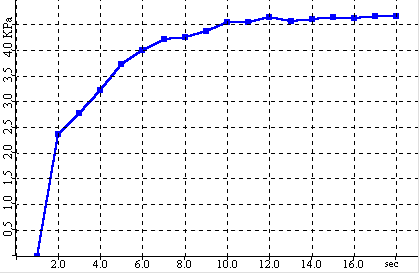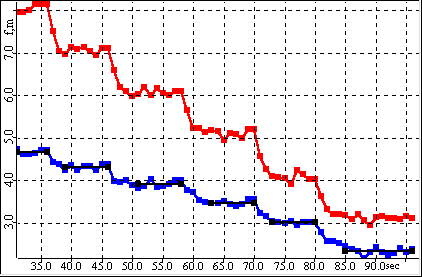# Wall Friction Analysis

The wall friction analysis is designed to determine the kinematic angle of surface friction for a sample material against a container material. This is achieved by measuring the friction force between the container material and the sample material under different loads to generate a wall yield locus. The analysis consists of three parts: consolidation, steady state and analysis. All parts are automatic.

In the consolidation step, the sample in the measurement cell is compressed to the preset starting load. With linear cells, this step includes twisting of the lid to help pack the material in the cell to what is called its “critical consolidation”. Critical consolidation is defined as the sample density at which it will reach a steady friction with minimal shear travel. This state in indicated by constant sample density or by a leveling off of the drop in normal load after each twist of the cell lid. For rotational cells, the consolidation step simply consists of compressing the sample until the normal load is reached.

 Sample Consolidation Normal Load versus TimeIn the steady state step, shear stress is applied to the sample until the measured friction force and sample volume become stable. With linear cells, the shear stress is applied by moving pushing the container material at a fixed rate relative to the upper ring. For rotational cells, the lid is rotated a fixed rate. The steady state point is the point at which the shear force becomes stable. At the steady state point, the sample has reached a repeatable, stable density relative to the applied compressive load.

 Steady State Shear Force versus TimeIn the analysis step, the friction force under the starting load is maintained until it is stable. The load on the sample is then reduced to a preset level and the friction force is again maintained until it is stable. This is repeated several times to produce a friction value for several applied loads.

 Friction Points Shear Force and Load vs TimeThe shear versus load data is then plotted to create a wall yield locus. A least squares regression is performed to calculate a linear function for the yield locus. The slope of the calculated line is the kinematic angle of surface friction.

 Friction Yield Locus Shear Force vs Normal Load